# Иностранная литература и современность: General Statistics: Ch 2 HW Flashcards

Set Details Share
created 9 years ago by GreenHero64
177,043 views
This course is designed to acquaint the student with the principles of descriptive and inferential statistics. Topics will include: types of data, frequency distributions and histograms, measures of central tendency, measures of variation, probability, probability distributions including binomial, normal probability and student's t distributions, standard scores, confidence intervals, hypothesis testing, correlation, and linear regression analysis. This course is open to any student interested in general statistics and it will include applications pertaining to students majoring in athletic training, pre-nursing and business.
updated 9 years ago by GreenHero64
College: First year, College: Second year, College: Third year, College: Fourth year
Subjects:
statistics, mathematics, probability & statistics
Page to share:
Embed this setcancel
COPY
code changes based on your size selection
Size:
X

1

After constructing a relative frequency distribution summarizing IQ scores of college​ students, what should be the sum of the relative​ frequencies?

If percentages are​ used, the sum should be​ 100%. If proportions are​ used, the sum should be 1.

2Identify the class​ width, class​ midpoints, and class boundaries for the given frequency distribution.

What is the class​ width?

What are the class​ midpoints?

What are the class​ boundaries?

3

51​, 54​, 57​, 60​, 63​, 66​, 69

49.5, 52.5, 58.5, 61.5, 64.5, 67.5, 70.5

3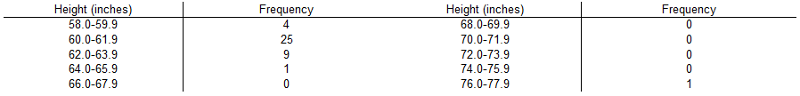Identify the class​ width, class​ midpoints, and class boundaries for the given frequency distribution.

What is the class​ width?

What are the class​ midpoints?

What are the class​ boundaries?

2

58.95, 60.95​, 62.95​, 64.95​, 66.95​, 68.95​, 70.95​, 72.95​, 74.95​, 76.95

57.95​, 59.95​, 61.95​, 63.95​, 65.95​, 67.95​, 69.95​, 71.95​, 73.95​, 75.95​, 77.95

4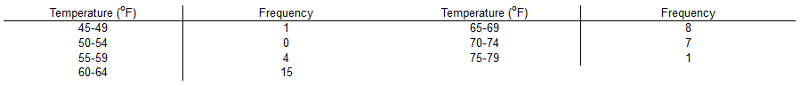Does the frequency distribution appear to have a normal distribution using a strict interpretation of the relevant​ criteria?

No, the distribution does not appear to be normal.

5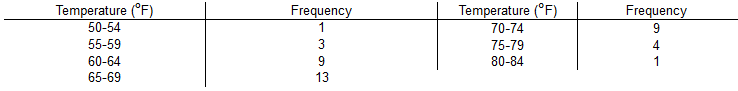Does the frequency distribution appear to have a normal​ distribution? Explain.

Yes, because the frequencies start​ low, proceed to one or two high​ frequencies, then decrease to a low​ frequency, and the distribution is approximately symmetric.

6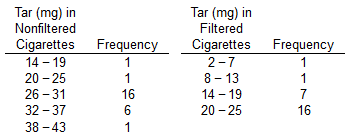Construct one table that includes relative frequencies based on the frequency distributions shown​ below, then compare the amounts of tar in nonfiltered and filtered cigarettes.

Do the cigarette filters appear to be​ effective?Yes, because the relative frequency of the higher tar classes is greater for nonfiltered cigarettes.

7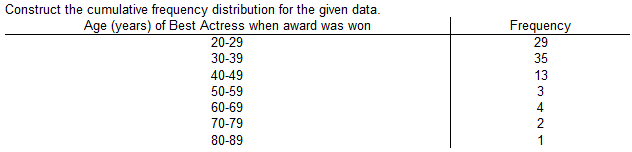Construct the cumulative frequency distribution for the given data.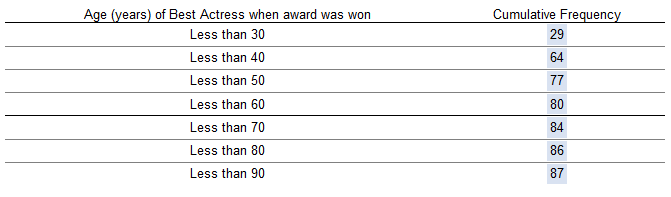8

Weights of statistics students were obtained by a teacher as part of an experiment conducted for the class. The last digit of those weights are listed below. Construct a frequency distribution with 10 classes.

0 0 0 0 0 0 0 0 0 1 2 2 3 4 4 4 5 5 5 5 5 5 5 5 5 6 6 8 8 9 9

Based on the​ distribution, do the weights appear to be reported or actually​ measured?

What can be said about the accuracy of the​ results?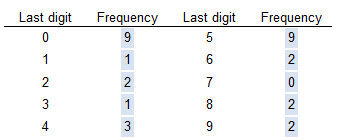The weights appear to be reported because there are disproportionately more 0s and 5s.

They are likely not very accurate because they appear to be reported.

9Refer to the accompanying data set and use the 25 home voltage measurements to construct a frequency distribution with five classes. Begin with a lower class limit of 121.1 ​volts, and use a class width of 0.2 volt.

Does the result appear to have a normal​ distribution? Why or why​ not?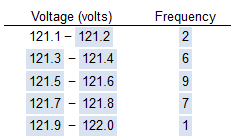Yes, because the frequencies start low, reach a maximum, then become low again, and are roughly symmetric about the maximum frequency.

10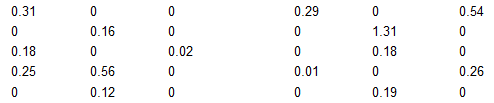The data represents the daily rainfall​ (in inches) for one month. Construct a frequency distribution beginning with a lower class limit of 0.00 and use a class width of 0.20.

Does the frequency distribution appear to be roughly a normal​ distribution?No, the distribution is not symmetric and the frequencies do not start off low.

11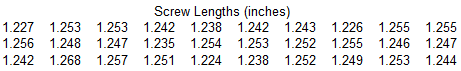Refer to the accompanying data set and use the 30 screw lengths to construct a frequency distribution. Begin with a lower class limit of 1.220 ​in, and use a class width of 0.010 in. The screws were labeled as having a length of 1 1/4 in.

Does the frequency distribution appear to be consistent with the​ label? Why or why​ not?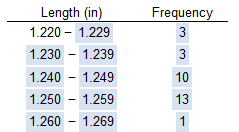Yes, because the frequencies are greatest in the closest to the labeled size.

12

Among fatal plane crashes that occurred during the past 65 ​years, 657 were due to pilot​ error, 71 were due to other human​ error, 613 were due to​ weather, 348 were due to mechanical​ problems, and 581 were due to sabotage.

What is the most serious threat to aviation​ safety, and can anything be done about​ it?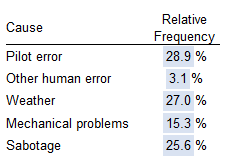Pilot error is the most serious threat to aviation safety. Pilots could be better trained.

13

Which characteristic of data is a measure of the amount that the data values​ vary?

Variation

14

Class width is found by​ _______.

subtracting a lower class limit from the next consecutive lower class limit

Class width is the difference between two consecutive lower class limits or two consecutive lower class boundaries in a frequency distribution.

15

The population of ages at inauguration of all U.S. Presidents who had professions in the military is​ 62, 46,​ 68, 64, 57.

Why does it not make sense to construct a histogram for this data​ set?

With a data set that is so​ small, the true nature of the distribution cannot be seen with a histogram.

16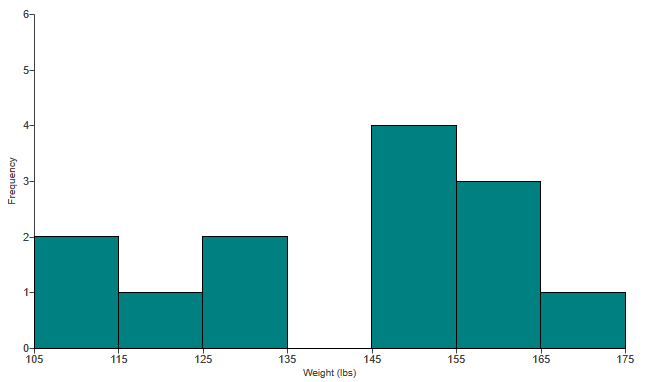The histogram to the right represents the weights​ (in pounds) of members of a certain​ high-school debate team.

What is the class​ width?

What are the approximate lower and upper class limits of the first​ class?

The class width is 10.

The approximate lower class limit is 105.The approximate upper class limit is 115.

17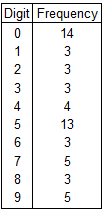The last digit of the heights of 56 statistics students were obtained as part of an experiment conducted for a class.

Use the frequency distribution to the right to construct a histogram.

What can be concluded from the distribution of the​ digits? Specifically, do the heights appear to be reported or actually​ measured?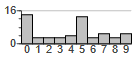The data appears to be reported. Certain heights occur a disproportionate number of times.

18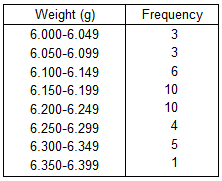The table below shows the frequency distribution of the weights​ (in grams) of​ pre-1964 quarters.

Use the frequency distribution to construct a histogram.

Does the histogram appear to depict data that have a normal​ distribution? Why or why​ not?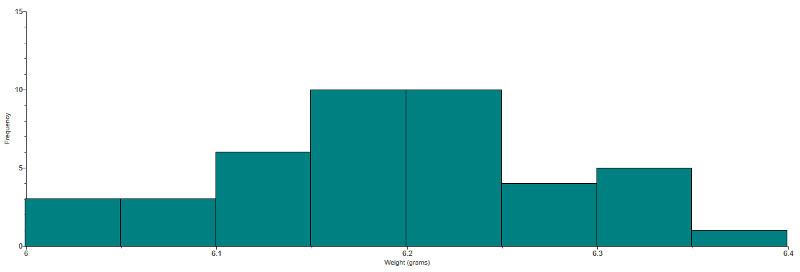The histogram appears to depict a normal distribution. The frequencies generally increase to a maximum and then​ decrease, and the histogram is roughly symmetric.

19

A bar chart and a Pareto chart both use bars to show frequencies of categories of categorical data. What characteristic distinguishes a Pareto chart from a bar​ chart, and how does that characteristic help us in understanding the​ data?

In a Pareto​ chart, the bars are always arranged in descending order according to frequencies. The Pareto chart helps us understand data by drawing attention to the more important​ categories, which have the highest frequencies.

A bar chart uses bars of equal width to show frequencies of categorical data. The vertical scale represents frequencies or relative frequencies. The horizontal scale identifies the different categories of qualitative data. When one wants a bar chart to draw attention to the more important​ categories, one can use a Pareto​ chart, which is a bar chart for categorical​ data, with the added stipulation that the bars are arranged in descending order according to frequencies. The bars decrease in height from left to right.

20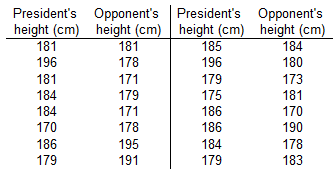The table provided below shows paired data for the heights of a certain​ country's presidents and their main opponents in the election campaign. Construct a scatterplot.

Does there appear to be a​ correlation?No, there does not appear to be a correlation because there is no general pattern to the data.

21Construct a scatter diagram using the data table to the right. This data is from a study comparing the amount of tar and carbon monoxide​ (CO) in cigarettes. Use tar for the horizontal scale and use carbon monoxide​ (CO) for the vertical scale.

Determine whether there appears to be a relationship between cigarette tar and CO.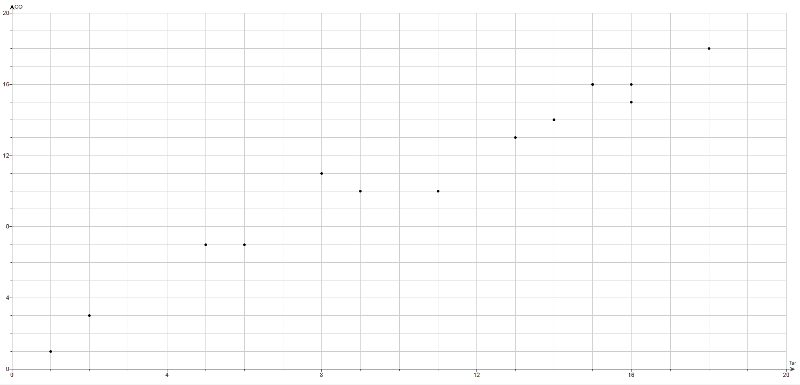Yes, as the amount of tar increases the amount of carbon monoxide also increases.

22Given below are the numbers of indoor movie​ theaters, listed in order by row for each year. Use the given data to construct a​ time-series graph.

What is the​ trend?

How does this trend compare to the trend for​ drive-in movie​ theaters?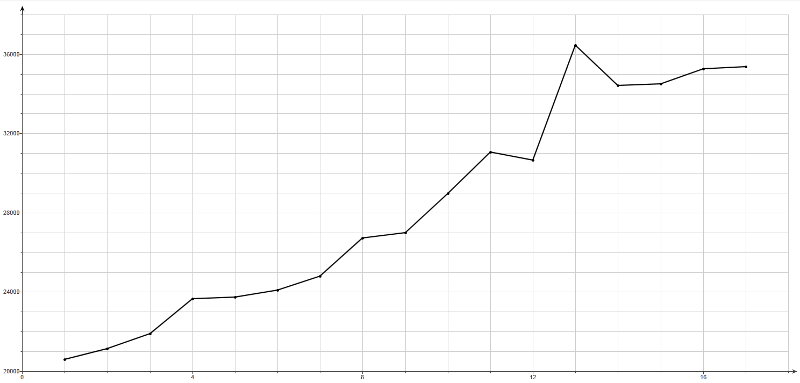There appears to be an upward​ trend, unlike​ drive-in movie​ theaters, which have a downward trend.

23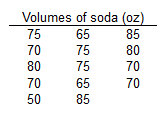The data table to the right represents the volumes of a generic soda brand.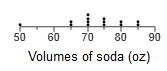Yes, the population appears to have a normal distribution because the dotplot resembles a​ "bell" shape.

When​ graphed, a normal distribution has an approximate​ "bell" shape. In a normal​ distribution, (1) the frequencies increase to a maximum and then​ decrease, and​ (2) the graph has​ symmetry, with the left half of the graph being roughly a mirror image of the right half.

Yes, the volume of 50 oz appears to be an outlier because it is far away from the other volumes.

Outliers are sample values that lie very far away from the vast majority of the other sample values.

24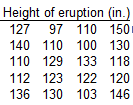The data represents the heights of eruptions by a geyser.

Use the heights to construct a stemplot. Is there strong evidence suggesting that the data are not from a population having a normal​ distribution?No, the distribution is not dramatically far from being a normal distribution with a​ "bell" shape, so there is not strong evidence against a normal distribution.

When​ plotted, a normal distribution has an approximate​ "bell" shape. In a normal​ distribution, the frequencies increase to a maximum and then​ decrease, and the distribution has​ symmetry, with the frequencies of the lower values being roughly a mirror image of the frequencies of the higher values.

25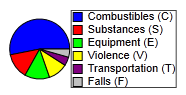In a recent​ year, 4386 people were killed while working. Here is a breakdown of​ causes: combustibles (2329); substances (605); equipment (590); violence (465); transportation ( 204); falls (193).

Use the data to construct a Pareto chart. Compare the Pareto chart to the pie chart.

Which graph is more effective in showing the relative importance of the causes of​ work-related deaths?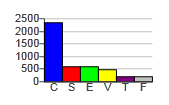A. The Pareto chart is better because it more clearly draws attention to the main cause of​ work-related death.

26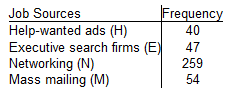A study was conducted to determine how people get jobs. The table lists data from 400 randomly selected subjects. Construct a Pareto chart that corresponds to the given data. If someone would like to get a​ job, what seems to be the most effective​ approach?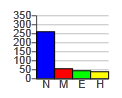Networking (N)

27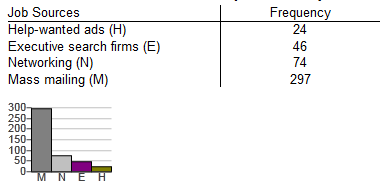A study was conducted to determine how people get jobs. The table below lists data from 400 randomly selected subjects.

Compare the pie chart found above to the Pareto chart given on the left. Can you determine which graph is more effective in showing the relative importance of job​ sources?The Pareto chart is more effective.

28The given data represent the number of people from a​ town, aged​ 25-64, who subscribe to a print magazine. Construct a frequency polygon.

Applying a loose interpretation of the requirements for a normal​ distribution, does the data appear to be normally​ distributed? Why or why​ not?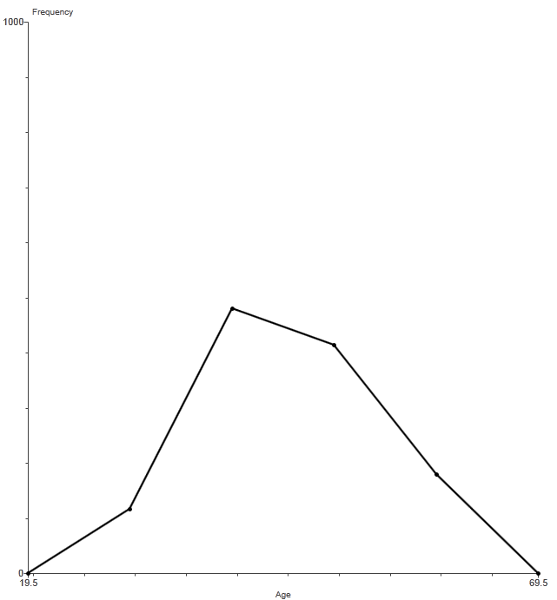The frequency polygon appears to roughly approximate a normal distribution because the frequencies increase to a​ maximum, then​ decrease, and the graph is roughly symmetric.

When​ graphed, a normal distribution has an approximate​ "bell" shape. In a normal​ distribution, the frequencies increase to a maximum and then​ decrease, and the distribution has​ symmetry, with the frequencies of the lower values being roughly a mirror image of the frequencies of the higher values.

29The given data represents a frequency distribution of the depth of recent earthquakes. Use the frequency distribution to construct a frequency polygon.

Applying a strict interpretation of the requirements for a normal​ distribution, do the depths appear to be normally​ distributed? Why or why​ not?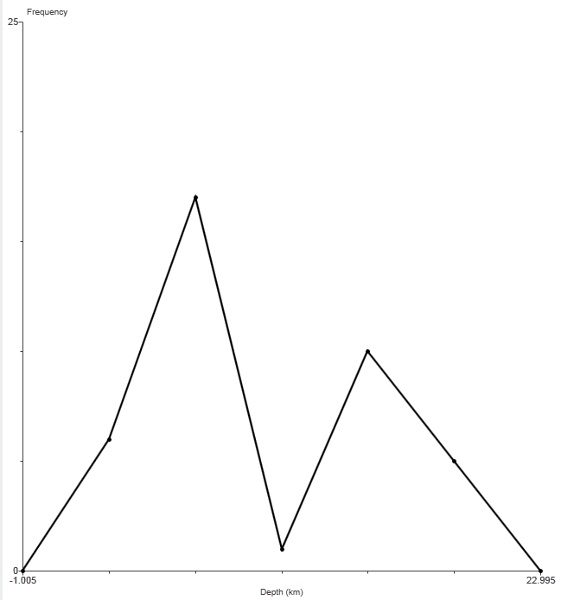The frequency polygon does not appear to approximate a normal distribution because the frequencies do not increase to a maximum and then​ decrease, and the graph is not symmetric.

When​ graphed, a normal distribution has an approximate​ "bell" shape. In a normal​ distribution, the frequencies increase to a maximum and then​ decrease, and the distribution has​ symmetry, with the frequencies of the lower values being roughly a mirror image of the frequencies of the higher values.

30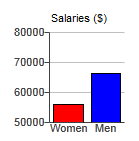The graph to the right compares teaching salaries of women and men at private colleges and universities.

What impression does the graph​ create?

Does the graph depict the data​ fairly? If​ not, construct a graph that depicts the data fairly.The graph creates the impression that men have salaries that are more than twice the salaries of women.

No, because the vertical scale does not start at zero.

31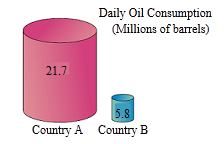The graph to the right uses cylinders to represent barrels of oil consumed by two countries.

Does the graph distort the data? Why or why​ not?

If the graph distorts the​ data, construct a graph that depicts the data fairly.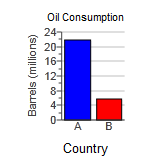​Yes, because the graph incorrectly uses objects of volume to represent the data.

32The graph to the right shows the braking distances for different cars measured under the same conditions.

Describe the ways in which this graph might be deceptive.

How much greater is the braking distance of Car A than the braking distance of Car​ C? Draw the graph in a way that depicts the data more fairly.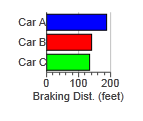By starting the horizontal axis at​ 100, the graph cuts off portions of the bars.

The braking distance of Car A is about 40​% greater than the braking distance of Car C.

33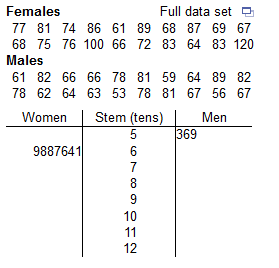A format for​ back-to-back stemplots representing the pulse rate of females and males from the given data is shown below. Complete the​ back-to-back stemplot, then compare the results.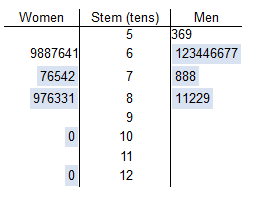The distribution of pulse rates for men is​ concentrated, centered around​ 60, whereas the distribution of pulse rates for women is more spread​ out, centered around 70.

34

Which of the following is NOT true about statistical​ graphs?

They utilize areas or volumes for data that are​ one-dimensional in nature.

Utilizing​ two- or​ three-dimensional pictures to represent​ one-dimensional data is poor practice and distorts the data.

35

Which of the following is a common distortion that occurs in​ graphs?

Using a​ two-dimensional object to represent data that are​ one-dimensional in nature

Using a​ two-dimensional object to represent data that are​ one-dimensional in nature is a bad practice that distorts the meaning of the data. When drawing such​ objects, artists can create false impressions that distort differences.

36